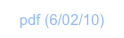Working with VectorsOverview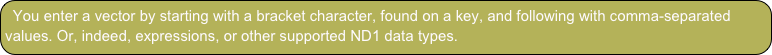There’s no limit to the number of elements (= members) in a vector, and you can mix and match data types. That includes expressions for vector elements, containing variables, function calls, etc.

If your vector contains only numbers or expressions we call it a “vector” (or “mathematical vector”).

Examples are:

[2, 4]                           a two-dimensional vector

[2, 6, 7]                       a three-dimensional vector

[(2, 4), i]                      a two-dimensional vector, containing complex numbers

[‘4-3’, ‘sin(a)’, 7]          a three-dimensional vector, containing expressions (w/ variables) for the first two elements

You can do math with a mathematical vector. That is, basic operators such as “+”, “-” will work.

If your vector is a mix of types, we tend to call it an array or list.

Examples are

[2, true, “Hi there”, ‘sin(x)*e^i’, “data:image/png;base64,iVBORhiugyu....”]

an array holding a variety of objects, incl. a screenshot

[“Hi”, [3,4,5], [[5,5],[4,6]]

an array holding a string, a vector, and a matrix

The “+” operator may or may not work with an array (depending on which objects “meet” with which objects in the two arrays you’re trying to add.

“List” is just another common name for arrays. We won’t be referring to it subsequently.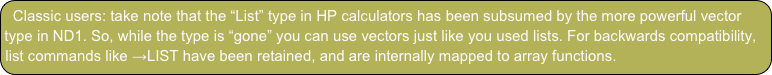Besides basic operators, vectors and arrays (and matrices) have their own menu with functions: Array

Some basic operations

Let’s exercise some vector functionality.

Type: 1, 2, 3

Enter

Tap 3

Tap →arrayWe created a three-dimensional vector.

Type: [4,5,6

Enter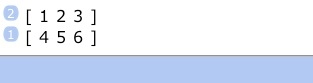And another.

Tap size (SIZE).

This returns the size of the vector and consumes it.

Undo. (Tap ⌫ (←))

Let’s exercise the cross product on those two vectors.

Go to the next menu page (tap → (NEXT)) and tap cross (CROSS).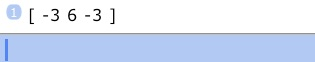Tap Enter to double the stack entry and tap ± (CHS) to negate it.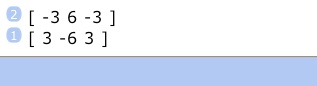Tap dot (DOT) to take the dot (scalar) product of the vector with its negated copy.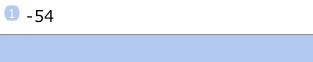There’s various more math functions that you can look up in the Functions Reference.

Let’s do a couple of things with an expression vector:

Type [‘x’,‘sin(π

Enter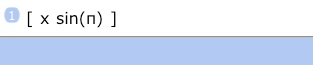Note, the missing closing characters are being added for you.

Also, note, commas and quotes are not shown. They’re still there but they’re not displayed to keep the stack look cleaner. If you edit the vector (⇩ (EDIT)), you’ll see the quotes and commas).

Duplicate that vector. (Press Enter.)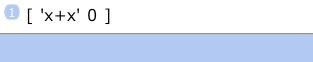We used a variable, x, in our expression vector. Because it was undefined (unless you have a variable ‘x’ in your current folder), x and x added to a new expression ‘x+x’. sin(π) + sin(π), on the other hand, were defined, evaluable expression which could be numerically added to yield the result 0.

There’s no limitations imposed on expressions as vector elements. And the plus operator will add matched-up elements just as they would be when individually added as stack items.

Finally, let’s do one operation on a mixed-object array.

Enter [5,”Hi”,(4,5(a real number, a string, a complex number)

Let’s extract the complex number out of this vector.

Tap “3”, tap get (GET) from the first page of the Array menu.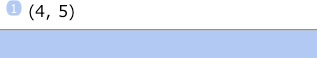As you can see, there are many functions in the Array menu that manipulate arrays. Please study the reference information to make sense of them. Some of the commands, such as the get (GET) function, are designed to be building blocks to be used in programming.

You should now have a good idea how vectors and arrays looks like, and how to use them in general.